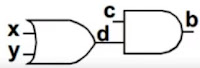### Modelling Sequential and Combinational Circuits: Part-1

SIMULATION AND SYNTHESIS:
• Simulation:
• Imitation of real IC which is generated by the Verilog code in a computer software called 'Simulator'. This is done in order to verify the IC design.
***The process of converting the Verilog code into an actual IC is very costly, and if there are problems found in the IC on a later stage then it will be a huge loss for the company. To avoid this, we use simulation and some other techniques.
• In simulation, we use different combinations of the program and see whether we are getting the output correct or not. If there is an issue, we need to keep making the changes until we get good health of the RTL design. Example Simulators used in the industry are:
• VCS - Synopsys
• ModelSim - Mentor Graphics
• NCSim (Inclusive Enterprise) - Cadence
• The term 'Simulation Time' is used to refer to the time value maintained by the simulator to model the actual time it would take for the system description being simulated.
• To fully support clear/predictable interactions, a single time slot is divided into multiple regions.
• Synthesis:
• Process of converting the Verilog code to an optimized gate level representation.
• The output is called netlist, which has only gates and their connecting nets (wires).
• Similar to writing a gate level Verilog code.
ASSIGN AND ALWAYS STATEMENTS:
• Assign: Continuous Assignment in System Verilog
• It is used to assign a wire with a value (used in Verilog).
• In System Verilog, a register can also be assigned with a value.
• A single block only has a single assign statement in it. So, there is no need of begin-end.
• It generates either a simple 'connection' or a simple combinational circuit./* The order of assign statement doesn't matter in the final circuit.*/
assign b=c&d;
assign d=x|y;
• Always (and Initial => initial statement is not synthesizable. ∴ It is used only in the Testbench code.): Procedural Assignment
• A single always block can have multiple statements within itself. Thus, it comes with a begin-end. 'Begin' and 'end' keywords identify a block of code associated with language constructs. It is somehow similar to opening and closing basis in "C".
CODE: always @(...sensitivity list...) begin
statement1;
statement2;
...
statementN;
end

• Even if there is a single statement in always block, it is a good practice to use begin-end thing.
Example:

always @ (x,y,z) begin;
y=x;
z=y;
end

always @ (x,y) begin;
if (x>y) begin
out=1;
end else begin
out=0;
end
end
• We can have the following synthesizable constructs in always ststement:
• if (similar use as in other programming language)
• if-else if (similar use as in other programming language)
• case (used like a statement)
• for (Testbench; careful use for synthesizable RTL code)
• while (Testbench; careful use for synthesizable RTL code)
• An "always" block can be used to represent a combinational logic or a sequential logic.
• TWO types of assignment in "always" block:
• Blocking assignment =
• Non-Blocking assignments <=
THE "ALWAYS" BLOCK - COMBINATIONAL LOGIC AND SEQUENTIAL LOGIC
• "always" block for combinational logic:
• Sensitivity list will have a list of variables that affects the combinational logic.
always @ (x,y,z) begin
x=y;
y=z;
end

OR

always @ (*) begin
x=y;
y=z;
end

> Here, '*' represents all the variables in the circuit.
• "always" block for sequential logic (the clock circuit)
• Sensitivity list will have only the clock signal @(posedge clk). There will be only one clk signal in the seq. ckt. using always block.
always @ (pasedge clk) begin
x=y;
y=z;
end
• In SV, three additional keywords were introduced to represent combinational logic: always_comb, always_latch and always_ff.
• It creates a complete sensitivity list by looking at the variables and nets that are read in the process, just like always '@*'.
• always_latch and always_ff are used for inferring transparent latches and flip-flops.
• Use "always" for sequential logic and "always_comb" for combinational logic.
• This will reduce the overhead of synthesizing tool and also synthesis tool can check the design intent.
Examples:

/******verilog******/

//always block for combinational logic
always @ (x,y,z) begin
y=x;
z=y;
.
.
.
end

//always block for sequential logic
always @ (posedge clk) begin
y=x;
z=y;
.
.
.
end

/******system verilog******/

//always block for combinational logic
always_comb begin
y=x;
z=y;
.
.
.
end

//always block for sequential logic
always @ (posedge clk) begin
y=x;
z=y;
.
.
.
end